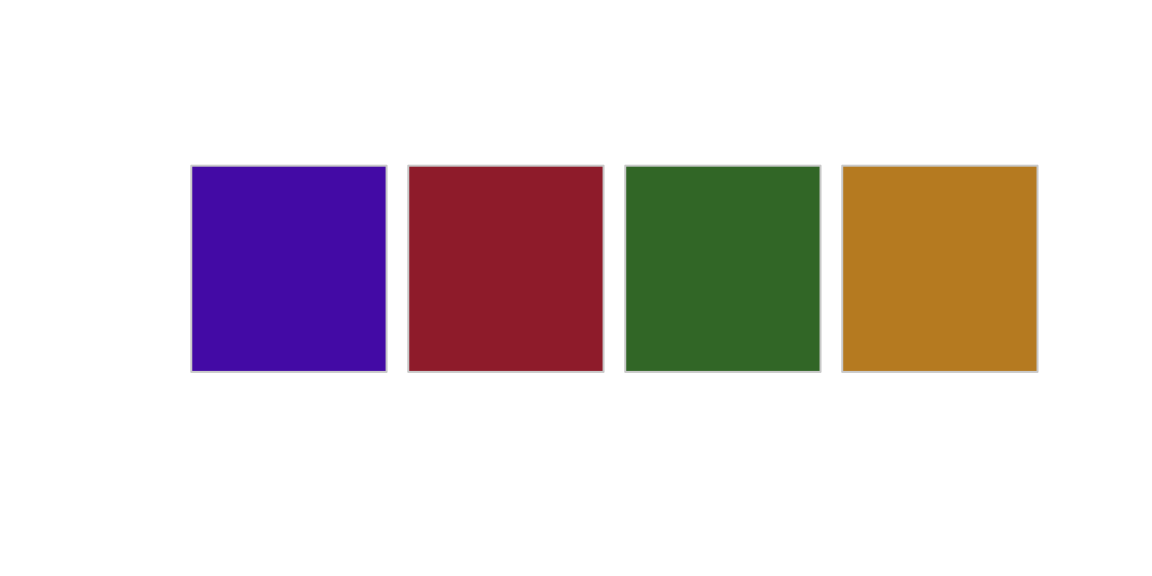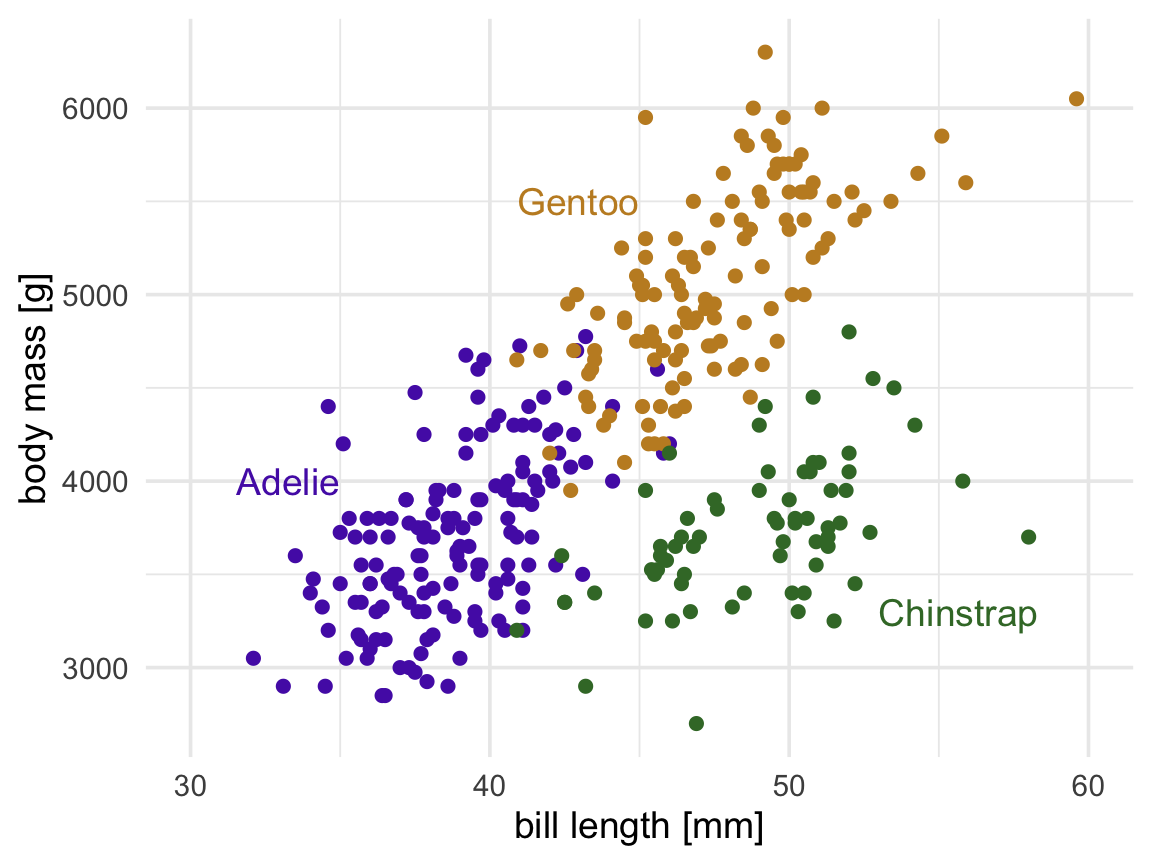Enter your name and EID here

This homework is due on April 26, 2021 at 11:00pm. Please submit as a pdf file on Canvas.

Problem 1: (2 pts)

Use the color picker app from the colorspace package (`colorspace::choose_color()`) to create a qualitative color scale containing four colors. One of the four colors should be `#5626B4`, so you need to find three additional colors that go with this one.

``````colors <- c("#5626B4", "#A12B37", "#3E7732", "#C38C29")

swatchplot(colors)``````Problem 2: (4 pts) Take the following scatter plot of the penguins dataset and make three modifications:

1. Use the colors you chose in Problem 1.
2. Improve the visual appearance by choosing a theme and cleaning up axis labels.
3. Remove the need for a legend by direct-labeling the points.
``````labels_data <- tibble(
species = c("Adelie", "Chinstrap", "Gentoo"),
bill_length_mm = c(35, 53, 45),
body_mass_g = c(4000, 3300, 5500),
hjust = c(1, 0, 1)
)

ggplot(penguins, aes(bill_length_mm, body_mass_g, color = species)) +
geom_point(size = 2, na.rm = TRUE) +
geom_text(
data = labels_data,
aes(label = species, hjust = hjust),
size = 14/.pt
) +
scale_x_continuous(
name = "bill length [mm]",
limits = c(30, 60)
) +
scale_y_continuous(
name = "body mass [g]"
) +
scale_color_manual(values = colors[c(1, 3, 4)], guide = "none") +
theme_minimal(14) ``````Problem 3: (4 pts) The following scatter plot shows per-capita income versus number of inhabitants in all Texas counties in 2010. Use `geom_text_repel()` to label a subset of the counties by name. You can choose the counties to subset as you wish. Also, choose a theme and clean up the axis labeling, and make any other improvements to the plot design you consider appropriate.

Hint: If you’re not sure how to select a subset of counties to label, check out the examples on the ggrepel website for some inspiration: https://ggrepel.slowkow.com/articles/examples.html#examples-1

``````tx_census <- read_csv("https://wilkelab.org/SDS375/datasets/US_census.csv") %>%
filter(state == "Texas") %>%
select(county = name, pop2010, per_capita_income)

set.seed(1234)

tx_census %>%
mutate(
# randomly label 20% as well as the most extreme caess
label = ifelse(
per_capita_income > 35000 |
pop2010 > 1e6,
county, ""
)
) %>%
ggplot(aes(pop2010, per_capita_income)) +
geom_point(size = 1.5, color = "#0072B2B0") +
geom_text_repel(
aes(label = label),
max.overlaps = Inf,
box.padding = .5,
force = 5,
size = 10/.pt
) +
scale_x_log10(
name = "number of inhabitants, 2010",
limits = c(1e1, 1e9)
) +
scale_y_continuous(
name = "per-capita income",
labels = scales::dollar_format(),
limits = c(8000, 45000)
) +
theme_bw(12)``````